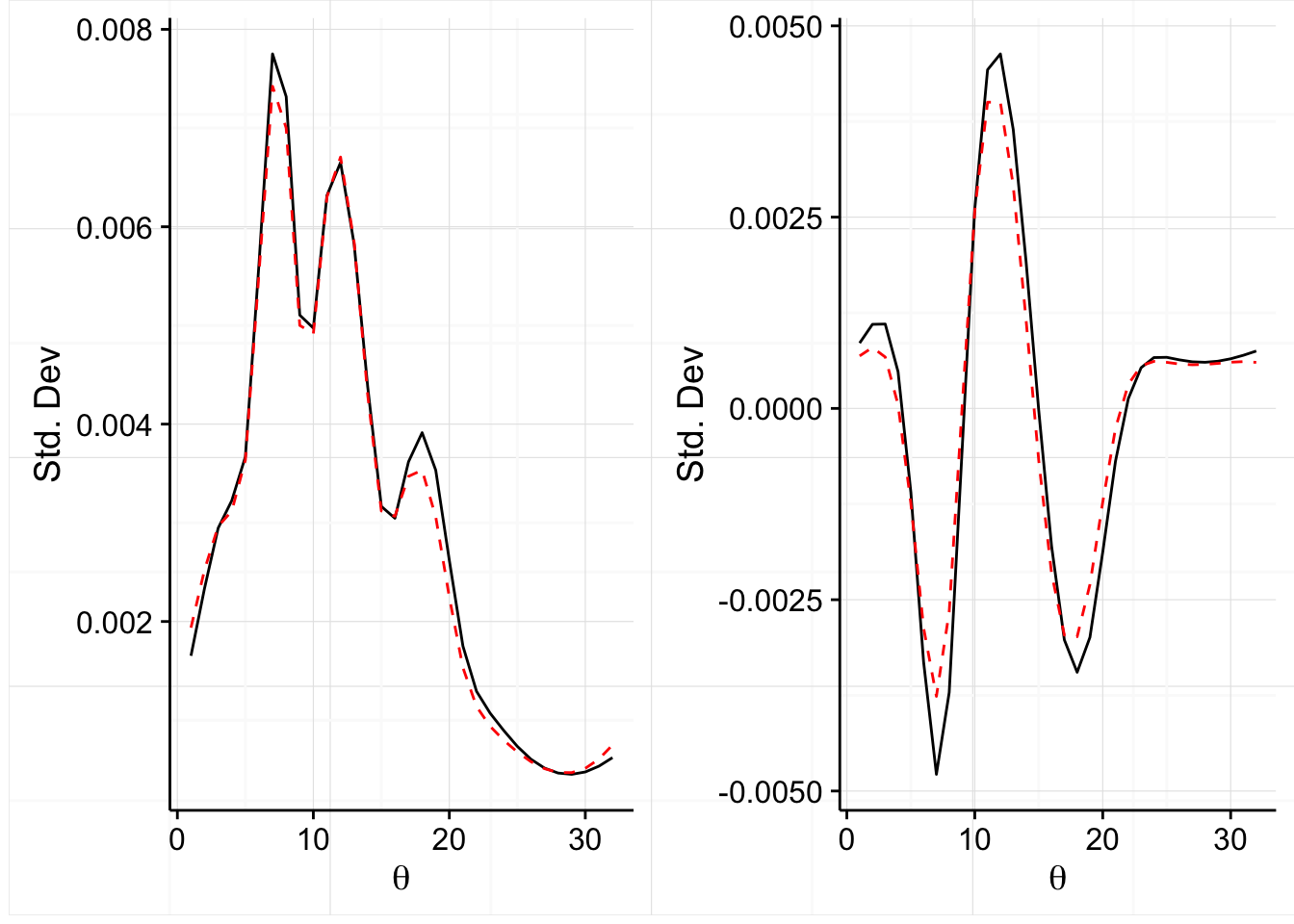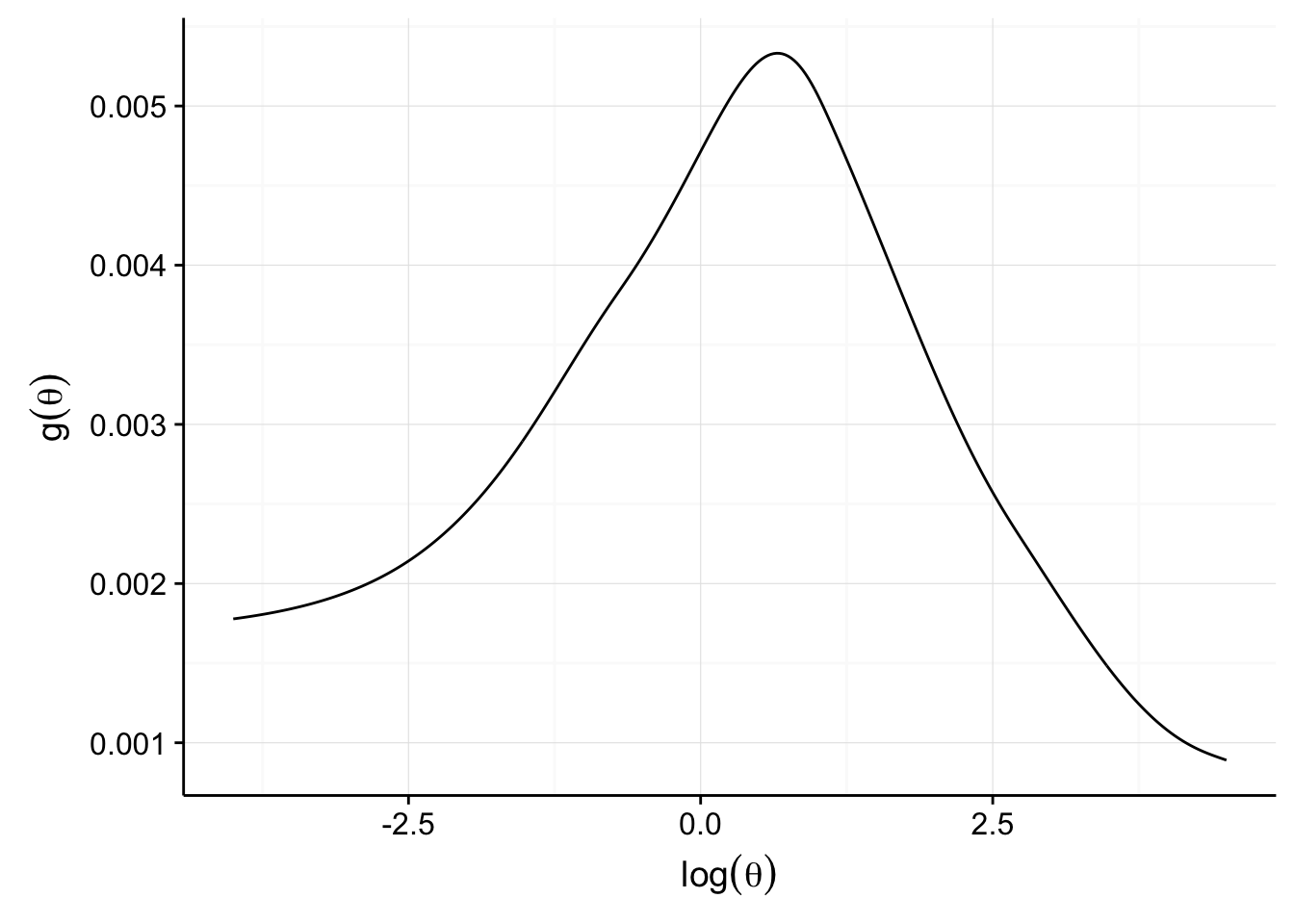## A simulation example

We start with a simulated Poisson example where the $$\Theta_i$$ are drawn from a chi-squared density with 10 degrees of freedom and the $$X_i|\Theta_i$$ are Poisson with expectation $$\Theta_i:$$

$\Theta_i \sim \chi^2_{10} \mbox{ and } X_i|\Theta_i \sim \mbox{Poisson}(\Theta_i)$

The $$\Theta_i$$ for this setting, with N = 1000 observations can be generated as follows.

set.seed(238923) ## for reproducibility
N <- 1000
Theta <- rchisq(N,  df = 10)

Next, the $$X_i|\Theta_i$$, for each of nSIM = 1000 simulations can be generated as below.

nSIM <- 1000
data <- sapply(seq_len(nSIM), function(x) rpois(n = N, lambda = Theta))

We take the discrete set $$\mathcal{T}=(1, 2, \ldots, 32)$$ as the $$\Theta$$-space and apply the deconv function in the package deconvolveR to estimate $$g(\theta).$$

library(deconvolveR)
tau <- seq(1, 32)
results <- apply(data, 2,
function(x) deconv(tau = tau, X = x, ignoreZero = FALSE,
c0 = 1))

The default setting for deconv uses the Poisson family and a natural cubic spline basis of degree 5 as $$Q.$$ The regularization parameter for this example (c0) is set to 1.

Some warnings are emitted by the nlm routine used for optimization, but they are mostly inconsequential.

Since deconv works on a sample at a time, the result above is a list of lists from which various statistics can be extracted. Below, we construct a table of values for various values of $$\Theta$$.

g <- sapply(results, function(x) x$stats[, "g"]) mean <- apply(g, 1, mean) SE.g <- sapply(results, function(x) x$stats[, "SE.g"])
sd <- apply(SE.g, 1, mean)
Bias.g <- sapply(results, function(x) x$stats[, "Bias.g"]) bias <- apply(Bias.g, 1, mean) gTheta <- pchisq(tau, df = 10) - pchisq(c(0, tau[-length(tau)]), df = 10) gTheta <- gTheta / sum(gTheta) simData <- data.frame(theta = tau, gTheta = gTheta, Mean = mean, StdDev = sd, Bias = bias, CoefVar = sd / mean) table1 <- transform(simData, gTheta = 100 * gTheta, Mean = 100 * Mean, StdDev = 100 * StdDev, Bias = 100 * Bias) The table below summarizes the results for some chosen values of $$\theta .$$ knitr::kable(table1[c(5, 10, 15, 20, 25), ], row.names=FALSE) theta gTheta Mean StdDev Bias CoefVar 5 5.6191465 5.4416722 0.3635378 -0.1235865 0.0668063 10 9.1646990 9.5316279 0.4917673 0.2588187 0.0515932 15 4.0946148 3.3421426 0.3119862 -0.0683187 0.0933492 20 1.1014405 0.9810001 0.2246999 -0.1225092 0.2290519 25 0.2255788 0.1496337 0.0677919 0.0602422 0.4530522 Although, the coefficient of variation of $$\hat{g}(\theta)$$ is still large, the $$g(\theta)$$ estimates are reasonable. We can compare the empirical standard deviations and biases of $$g(\hat{\alpha})$$ with the approximation given by the formulas in the paper. library(ggplot2) library(cowplot) theme_set(theme_get() + theme(panel.grid.major = element_line(colour = "gray90", size = 0.2), panel.grid.minor = element_line(colour = "gray98", size = 0.5))) p1 <- ggplot(data = as.data.frame(results[]$stats)) +
geom_line(mapping = aes(x = theta, y = SE.g), color = "black", linetype = "solid") +
geom_line(mapping = aes(x = simData$theta, y = simData$StdDev), color = "red", linetype = "dashed") +
labs(x = expression(theta), y = "Std. Dev")

p2 <- ggplot(data = as.data.frame(results[]$stats)) + geom_line(mapping = aes(x = theta, y = Bias.g), color = "black", linetype = "solid") + geom_line(mapping = aes(x = simData$theta, y = simData$Bias), color = "red", linetype = "dashed") + labs(x = expression(theta), y = "Std. Dev") plot_grid(plotlist = list(p1, p2), ncol = 2)The approximation is quite good for the standard deviations, but a little too small for the biases. ## The Shakespeare data Here we are given the word counts for the entire Shakespeare canon in the data set bardWordCount. We assume the $$i$$th distinct word appeared $$X_i \sim Poisson(\Theta_i)$$ times in the canon. data(bardWordCount) str(bardWordCount) ## num [1:100] 14376 4343 2292 1463 1043 ... We take the support set $$\mathcal{T}$$ for $$\Theta$$ to be equally spaced on the log-scale and the sample space for $$\mathcal{X}$$ to be $$(1,2,\ldots,100).$$ lambda <- seq(-4, 4.5, .025) tau <- exp(lambda) Using a regularization parameter of c0=2 we can deconvolve the data to get $$\hat{g}.$$ result <- deconv(tau = tau, y = bardWordCount, n = 100, c0=2) stats <- result$stats

The plot below shows the Empirical Bayes deconvoluation estimates for the Shakerspeare word counts.

ggplot() +
geom_line(mapping = aes(x = lambda, y = stats[, "g"])) +
labs(x = expression(log(theta)), y = expression(g(theta)))The quantity $$R(\alpha)$$ in the paper (Efron, Biometrika 2015) can be extracted from the stats list; in this case for a regularization parameter of c0=2 we can print its value:

print(result\$R)
## NULL

The stats list contains other estimates quantities as well.

As noted in the paper citing this package, about 44 percent of the total mass of $$\hat{g}$$ lies below $$\Theta = 1$$, which is an underestimate. This can be corrected for by defining $\tilde{g} = c_1\hat{g} / (1 - e^{-\theta_j}),$ where $$c_1$$ is the constant that normalizes $$\tilde{g}$$.

gt <- stats[, "g"] / (1 - exp(-tau))
gt <- gt / sum(gt)
d <- data.frame(lambda = lambda, g = stats[, "g"], SE.g = stats[, "SE.g"])
indices <- seq(1, length(lambda), 5)
ggplot(data = d) +
geom_line(mapping = aes(x = lambda, y = g)) +
geom_errorbar(data = d[indices, ],
mapping = aes(x = lambda, ymin = g - SE.g, ymax = g + SE.g),
width = .01, color = "blue") +
labs(x = expression(log(theta)), y = expression(g(theta))) +
ylim(0, 0.006) +
geom_line(mapping = aes(x = lambda, y = gt), linetype = "dashed", color = "red")